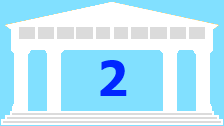# Advanced Problem Solving Module 2### Advanced Problem Solving Module 2

This module is about logical thinking and mathematical notation. Mathematicians use lots of notation and shorthand when writing mathematics and it's good to get into the habit of using the correct notation when writing your own mathematics. Logical thinking is at the heart of mathematics, and it's important to have a clear grasp of logical ideas when you are embarking on advanced mathematics examinations.

### Notes on Logic

##### Age 16 to 18Challenge Level
Some notes to describe the words and symbols which occur in logic and mathematics

### Some Useful Mathematical Notation

##### Age 16 to 18
Here is a glossary of common notation used in higher mathematics.

### Iffy Logic

##### Age 14 to 18Challenge Level
Can you rearrange the cards to make a series of correct mathematical statements?

### Mind Your Ps and Qs

##### Age 16 to 18Challenge Level
Sort these mathematical propositions into a series of 8 correct statements.